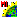# vpoly.sml

###Download

#### Syntax Highlighing:

```comments, key words, predefined symbols, class members & methods, functions & classes
```
```# vpoly.sml
clear();
vector V;
array numeric xPoints, yPoints, polyList;
numeric numPolys, polyNum, polyCount;
GetOutputVector(V, "VectorToolkit");
# draw some boxes  - this will create polygons
# all closed lines are converted to polygons
xPoints = 0;
yPoints = 0;
xPoints = 100;
yPoints = 0;
xPoints = 100;
yPoints = 100;
xPoints = 0;
yPoints = 100;
xPoints = 0;
yPoints = 0;
numeric i, j;
for i = 0 to 5 {
VectorAddLine(V, 5, xPoints, yPoints);
for j = 1 to 5 {
xPoints[j] = xPoints[j] + 150;
}
}
numPolys = NumVectorPolys(V);
print("number of polygons: ", numPolys);
# now delete one polygon
polyNum = 1;
VectorDeletePoly(V, polyNum);
numPolys = NumVectorPolys(V);
print("number of polygons: ", numPolys);
# now delete multiple polygons
polyList = 1;
polyList = 3;
polyCount = 2;
VectorDeletePolys(V, polyList, polyCount);
numPolys = NumVectorPolys(V);
print("number of polygons: ", numPolys);
```